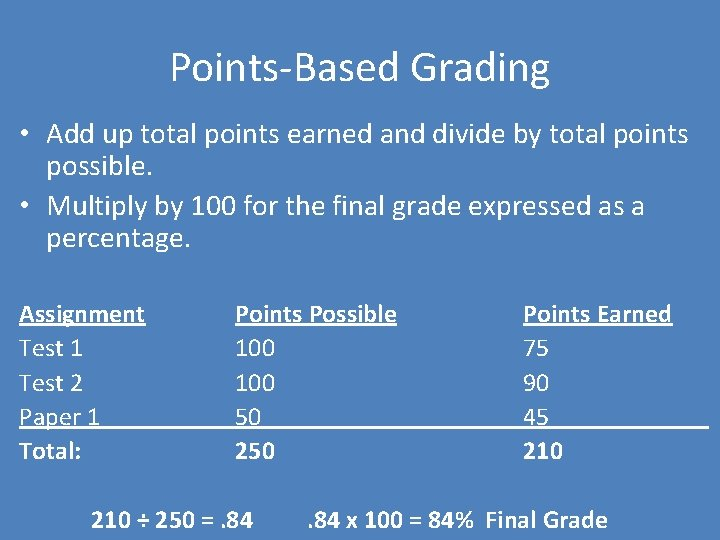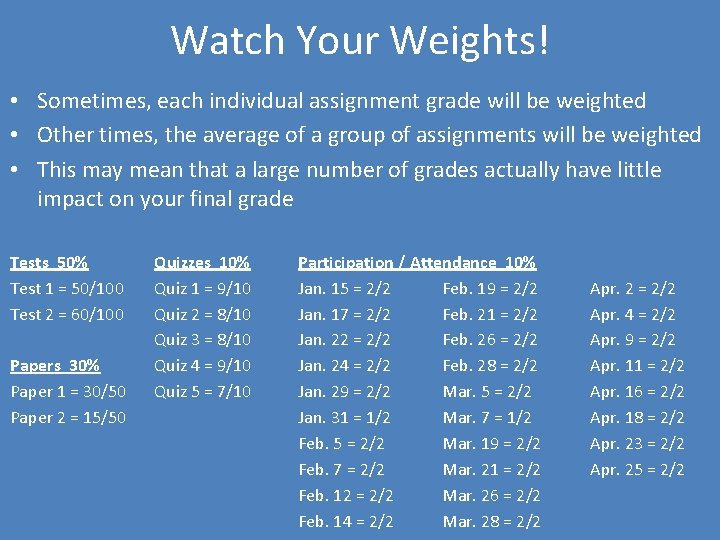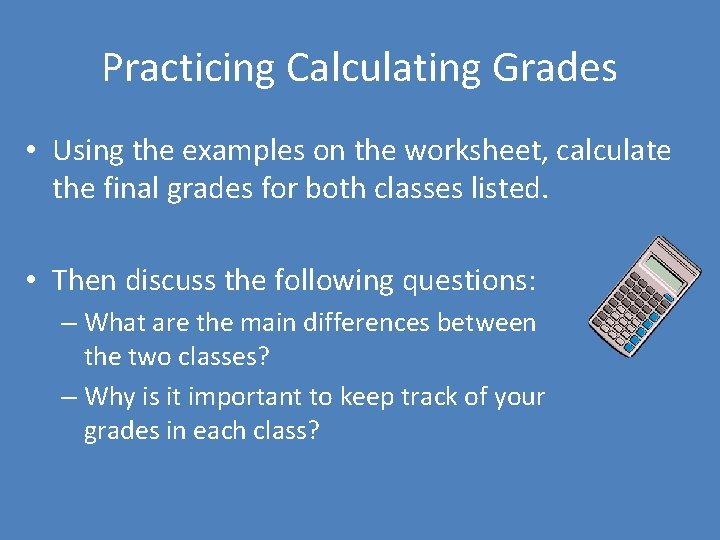# Calculating Grades in College Classes College Grading Calculating

• Slides: 10
Download presentationCalculating Grades in College ClassesCollege Grading • Calculating your grade in college courses can sometimes seem challenging • Each course may have a different configuration of tests and assignments, with each weighted differently in your final grade • However, professors usually outline their grading structure in the course syllabusCollege Grading • Some courses may weight assignments by percentage For example… • Test 1 is worth 40% of your grade • Test 2 is worth 40% of your grade • Paper 1 is worth 20% of your grade • Others may use a points system For example… • Test 1 is worth 100 points • Test 2 is worth 100 points • Paper 1 is worth 50 pointsPoints-Based Grading • Add up total points earned and divide by total points possible. • Multiply by 100 for the final grade expressed as a percentage. Assignment Test 1 Test 2 Paper 1 Total: Points Possible 100 50 210 ÷ 250 =. 84 Points Earned 75 90 45 210 . 84 x 100 = 84% Final GradePercentage-Based Grading • For each assignment, divide points earned by points possible. Multiply by 100 for the assignment grade expressed as a percentage. • Then multiply each assignment grade by the percentage that specific assignment is weighted in the total grade. • Last, add up all the weighted grades to get the final grade expressed as a percentage. Assignment Points Possible Points Earned Test 1 40 30 Test 2 80 Paper 1 10 Calculations of Grade Earned % Weight Calculations of Weighted Grade 30 ÷ 40 =. 75 x 100 = 75 40% 75 x. 40 = 30 72 72 ÷ 80 =. 9 x 100 = 90 40% 90 x. 40 = 36 9 9 ÷ 10 =. 9 x 100 = 90 20% 90 x. 20 = 18 30 + 36 + 18 = 84 84% Final GradeWatch Your Weights! • Sometimes, each individual assignment grade will be weighted • Other times, the average of a group of assignments will be weighted • This may mean that a large number of grades actually have little impact on your final grade Tests 50% Test 1 = 50/100 Test 2 = 60/100 Papers 30% Paper 1 = 30/50 Paper 2 = 15/50 Quizzes 10% Quiz 1 = 9/10 Quiz 2 = 8/10 Quiz 3 = 8/10 Quiz 4 = 9/10 Quiz 5 = 7/10 Participation / Attendance 10% Jan. 15 = 2/2 Feb. 19 = 2/2 Jan. 17 = 2/2 Feb. 21 = 2/2 Jan. 22 = 2/2 Feb. 26 = 2/2 Jan. 24 = 2/2 Feb. 28 = 2/2 Jan. 29 = 2/2 Mar. 5 = 2/2 Jan. 31 = 1/2 Mar. 7 = 1/2 Feb. 5 = 2/2 Mar. 19 = 2/2 Feb. 7 = 2/2 Mar. 21 = 2/2 Feb. 12 = 2/2 Mar. 26 = 2/2 Feb. 14 = 2/2 Mar. 28 = 2/2 Apr. 2 = 2/2 Apr. 4 = 2/2 Apr. 9 = 2/2 Apr. 11 = 2/2 Apr. 16 = 2/2 Apr. 18 = 2/2 Apr. 23 = 2/2 Apr. 25 = 2/2Watch Your Weights! Assignment Points Possible Points Earned Tests 200 110 Papers 100 Quizzes Participation /Attendance Calculations of Grade Earned % Weight Calculations of Weighted Grade 110 ÷ 200 =. 55 x 100 = 55 50% 55 x. 50 = 27. 5 45 45 ÷ 100 =. 45 x 100 = 45 30% 45 x. 30 = 13. 5 50 41 41 ÷ 50 =. 82 x 100 = 82 10% 82 x. 10 = 8. 2 56 54 54 ÷ 56 =. 96 x 100 = 96 10% 96 x. 10 = 9. 6 27. 5 + 13. 5 + 8. 2 + 9. 6 = 58. 8 59% Final Grade • Most (80%) of a student’s final grade in this course is based on grades on the tests and papers • Even though there are many more grades in the categories of quizzes and participation/attendance, all of those together are only worth 20%Tips for Watching Your Weights • Pay careful attention to the weights of assignments in calculating your overall grade. – Find this information in your syllabus for each course – If it’s not listed, ask your professor • Use this information to prioritize and manage your time. – Give the most attention to assignments that have the most impact on your grade – e. g. , spend the time to revise your paper (15% of grade) before taking an online quiz (2% of your grade)Practicing Calculating Grades • Using the examples on the worksheet, calculate the final grades for both classes listed. • Then discuss the following questions: – What are the main differences between the two classes? – Why is it important to keep track of your grades in each class?This work is licensed under a Creative Commons Attribution-Non. Commercial 3. 0 Unported License.